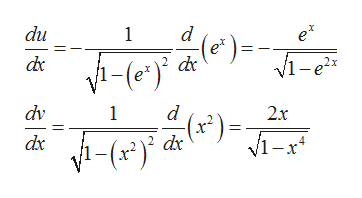# 2. Calculate the derivative of y = arccos(earcsin(x2)

Question
check_circleExpert Solution
Step 1

Let u=arcacos(e^x) and v=arcsin(x^2), then y=u/v

Step 2

Then , we find du/dx and dv/dx by using result that derivative of arcinx with respect to x is  1/ sqrt(1-x^2) and that of arccosx is -1/ sqrt(1-x^2)help_outlineImage Transcriptionclosedu d 1 e 2 dx V1-e2 x 1-(e dv 1 d ) 2.x 2 dx dx vi-x* fullscreen
Step 3

Now qotient rule of differenti...

### Want to see the full answer?

See Solution

#### Want to see this answer and more?

Solutions are written by subject experts who are available 24/7. Questions are typically answered within 1 hour*

See Solution
*Response times may vary by subject and question
Tagged in

### Derivative